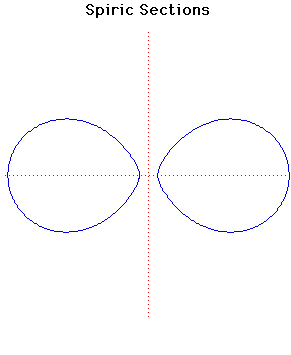# Curves

### Spiric SectionsCartesian equation:
$(r^{2} - a^{2} + c^{2} + x^{2} + y^{2})^{2} = 4r^{2}(x^{2} + c^{2})$

### Description

After Menaechmus constructed conic sections by cutting a cone by a plane, around 150 BC which was 200 years later, the Greek mathematician Perseus investigated the curves obtained by cutting a torus by a plane which is parallel to the line through the centre of the hole of the torus.

In the formula of the curve given above the torus is formed from a circle of radius $a$ whose centre is rotated along a circle of radius $r$. The value of $c$ gives the distance of the cutting plane from the centre of the torus.

When$c = 0$ the curve consists of two circles of radius $a$ whose centres are at $(r, 0)$ and $(-r, 0)$.

If $c = r + a$ the curve consists of one point, namely the origin, while if $c > r + a$ no point lies on the curve.

### Associated Curves

Definitions of the Associated curves